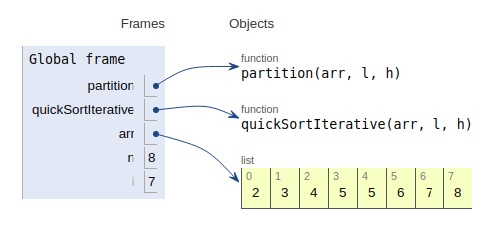# Python Program for Iterative Quick Sort

Problem statement − We are given an array, we need to sort it using the concept of quick sort using iterative way

Here we first partition the array and sort the separate partition to get the sorted array.

Now let’s observe the solution in the implementation below−

## Example

Live Demo

# iterative way
def partition(arr,l,h):
i = ( l - 1 )
x = arr[h]
for j in range(l , h):
if arr[j] <= x:
# increment
i = i+1
arr[i],arr[j] = arr[j],arr[i]
arr[i+1],arr[h] = arr[h],arr[i+1]
return (i+1)
# sort
def quickSortIterative(arr,l,h):
# Creation of a stack
size = h - l + 1
stack =  * (size)
# initialization
top = -1
# push initial values
top = top + 1
stack[top] = l
top = top + 1
stack[top] = h
# pop from stack
while top >= 0:
# Pop
h = stack[top]
top = top - 1
l = stack[top]
top = top - 1
# Set pivot element at its correct position
p = partition( arr, l, h )
# elements on the left
if p-1 > l:
top = top + 1
stack[top] = l
top = top + 1
stack[top] = p - 1
# elements on the right
if p+1 < h:
top = top + 1
stack[top] = p + 1
top = top + 1
stack[top] = h
# main
arr = [2,5,3,8,6,5,4,7]
n = len(arr)
quickSortIterative(arr, 0, n-1)
print ("Sorted array is:")
for i in range(n):
print (arr[i],end=" ")

## Output

Sorted array is
2 3 4 5 5 6 7 8All the variables are declared in the local scope and their references are seen in the figure above.

## Conclusion

In this article, we have learned about how we can make a Python Program for Iterative Quick Sort.